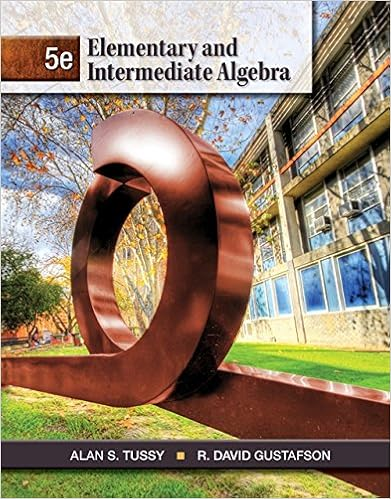# T z t 2 1 1 u 2 u du find f 00 3 12 find the

• Notes
• 7
• 100% (1) 1 out of 1 people found this document helpful

This preview shows page 2 - 4 out of 7 pages.

##### We have textbook solutions for you!
The document you are viewing contains questions related to this textbook.The document you are viewing contains questions related to this textbook.
Chapter 9 / Exercise 17
Elementary and Intermediate Algebra
Tussy/GustafsonExpert Verified
t ) = Z t 2 1 1 + u 2 u du , find F 00 (3).
(12) Find the interval(s) on which the curve y = Z x 0 dt 1 + t + t 2
##### We have textbook solutions for you!
The document you are viewing contains questions related to this textbook.The document you are viewing contains questions related to this textbook.
Chapter 9 / Exercise 17
Elementary and Intermediate Algebra
Tussy/GustafsonExpert Verified
Your signature: (13) Let f and g be two continuous functions. Among the following three statements, the correct one(s) is/are . (If all wrong, write NONE.) (a) Z b a [ f ( x ) g ( x )] dx = Z b a f ( x ) dx · Z b a g ( x ) dx (b) Z b a f ( x 2 ) dx = Z b a [ f ( x )] 2 dx (c) Z b a [ f ( x )] 2 dx = Z b a f ( x ) dx 2 Hints for Q13: 1. Would your answer change if you were told that f ( x ) = g ( x ), for all x ? 2. Would your answer change if you were told that a = 0, b = 1, and the graph of f ( x ) is a horizontal line passing through (0 , 1)? 3. Would your answer change if you were told that a = 0, b = 1, and the graph of f ( x ) is a horizontal line passing through (0 , - 1)? 4. Would your answer change if you were told that a = 0, b = 2, and the graph of f ( x ) is a horizontal line passing through (0 , 1)? (14) Given the graph of the function g as below. Let G ( x ) = Z x 0 g ( t ) dt . (a) G (5) = , and the average value of g on [0 , 5] is . (b) In the open interval (0 , 5), G ( t ) has critical point(s) at x = . (c) In the open interval (0 , 5), G ( t ) has inflection point(s) at approximately x = (d) In the open interval (0 , 5), G ( t ) has local max at x = , and the value of the function at that point is . (e) G ( t ) is increasing and concave up in the interval(s) . (15) Use the Second Fundamental Theorem of Calculus to find: (a) d dx Z x 0 ln( t ) dt = (b) d dx " Z x 2 - x 2 e t 2 dt # = (c) d dx Z x π t 2 sin( t 2 ) dt = (d) d dx Z x
•••